# How to search for value in all tabs in Excel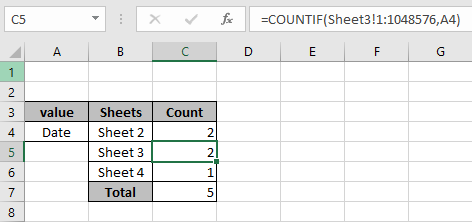In this article, we will learn about how to search for value in all tabs in Excel in Excel.

Scenario:

When working with data along different tabs of the current workbook. We need to search for any particular text or number value in the worksheet. COUNTIF counts the number of values in range. But the problem is when you use the formula in the same sheet you are finding the value, the Excel shows an error message : "There are one or more circular references where a formula refers to  its own cell either directly or indirectly. This might cause them to calculate incorrectly. Try removing or changing these references, or moving the formulas to different cells."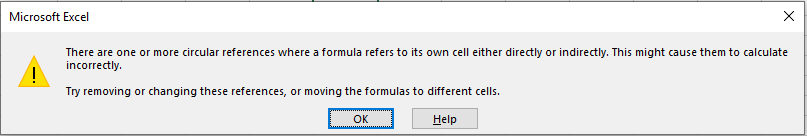So now for this formula we will add a new sheet or tab in the current workbook and do all the operations in the new sheet. And counter all the sheets one by one. Here for the purpose consider a new sheet "Count" for the count.

COUNTIF counts the number of values in range. We will use the range as the whole sheet. First we count the value for one sheet.

Syntax to find max with multiple criteria

=COUNTIF(Sheet_num!1:1048576,value)

Sheet_num : name of sheet to search.

1:1048576 : number of cell from top left to the right bottom.

value : value to look for.

Note : Use Ctrl + Shift + arrow ( ^ > < v ) keys to move along the sheet swiftly, instead of selecting range using mouse pointers.

Example:

All of these might be confusing to understand. So, let's test this formula via running it on the example shown below. Here we will perform the formula over values with criteria.

Use the formula:

=COUNTIF(Sheet2!1:1048576,A4)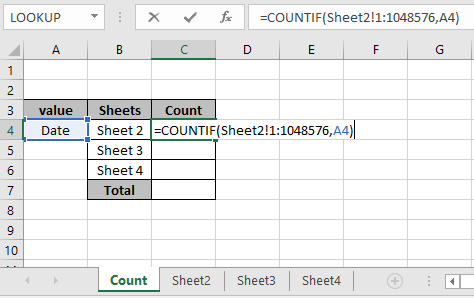Here we matched the range (1:1048576) in Sheet2 with value "date" in A4 cell. Press Enter to get the count in sheet2.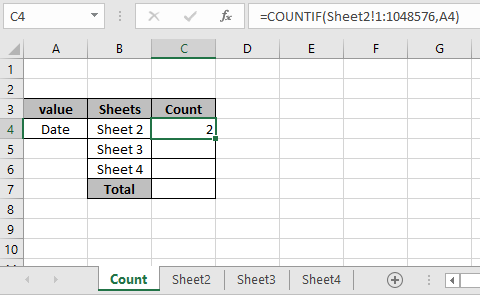As you can see we have the count of value "Date" in Sheet2 comes out to be 2. Similarly we can get the count of each workbook by freezing the value cell and changing the sheet names.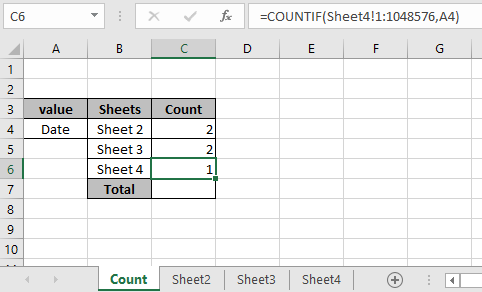Here is the count of the value in all the sheets named. Get the SUM of all the counts to get the count of value in all sheets named.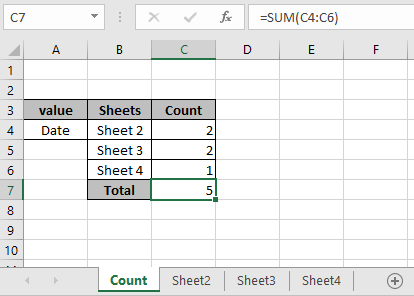There are 5 "Date" value in the workbook. We can get the

Use one formula to calculate all the count in workbook.

=COUNTIF(Sheet2!1:1048576,A4)+COUNTIF(Sheet3!1:1048576,A4)+COUNTIF(Sheet4!1:1048576,A4)

Here are some observational notes using the above formula.

Notes:

1. This formula counts all the values in the workbook.
2. Donot use the formula in the same sheet which is also used for the range.
3. Named range in the formula be used with correct keywords.

Hope this article about How to search for value in all tabs in Excel is explanatory. Find more articles on reference formulas here. If you liked our blogs, share it with your fristarts on Facebook. And also you can follow us on Twitter and Facebook. We would love to hear from you, do let us know how we can improve, complement or innovate our work and make it better for you. Write to us at info@exceltip.com.

Related Articles

COUNTIFS with Dynamic Criteria Range: Count cells having dynamic criteria range using COUNTIFS function.

COUNTIFS Two Criteria Match: Count cells having multiple criteria range using COUNTIFS function.

COUNTIFS With OR For Multiple Criteria: Count cells using the logic functions with COUNTIFS function.

The COUNTIFS Function in Excel: use of COUNTIFS function to count cells having multiple criteria

How to Use Countif in VBA in Microsoft Excel: Use the COUNTIF function in VBA to count cells.

How to use wildcards in excel: catch phrases and keywords using the explained wildcard characters in excel

Popular Articles

50 Excel Shortcut to Increase Your Productivity : Get faster at your task. These 50 shortcuts will make you work even faster on Excel.

The VLOOKUP Function in Excel : This is one of the most used and popular functions of excel that is used to lookup value from different ranges and sheets.

COUNTIF in Excel 2016 : Count values with conditions using this amazing function. You don't need to filter your data to count specific values. Countif function is essential to prepare your dashboard.

How to Use SUMIF Function in Excel : This is another dashboard essential function. This helps you sum up values on specific conditions.

Terms and Conditions of use

The applications/code on this site are distributed as is and without warranties or liability. In no event shall the owner of the copyrights, or the authors of the applications/code be liable for any loss of profit, any problems or any damage resulting from the use or evaluation of the applications/code.